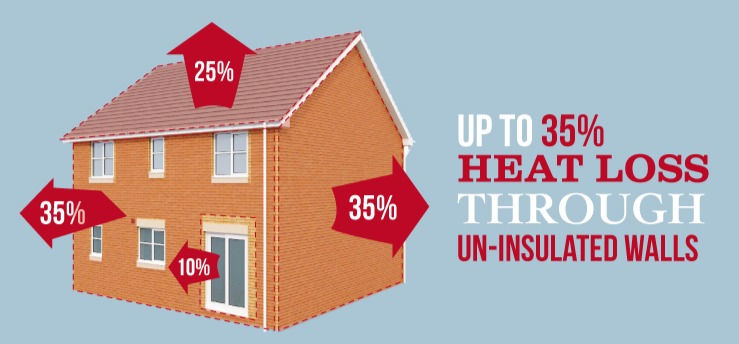top of page
• James Flemington

# Why we all should have a Heat Loss Survey prior to a new Boiler

Updated: May 10

Heat loss calculation is an important aspect of domestic property design and renovation. It helps to determine the amount of heat required to maintain a comfortable living environment, which in turn influences the choice of heating system and energy efficiency measures. In this blog post, we will discuss the basics of heat loss calculation for domestic properties.

What is Heat Loss?Heat Loss Survey

Heat loss is the amount of heat energy that escapes from a building through the walls, roof, windows, doors, and other openings. Heat loss can occur in various ways, such as conduction, convection, and radiation. Conduction is the transfer of heat through solid materials, while convection is the transfer of heat through fluids like air. Radiation is the transfer of heat through electromagnetic waves.

Why is Heat Loss Calculation Important?

Heat loss calculation is important because it helps to determine the heating requirements of a building. By knowing the amount of heat lost from a building, we can determine the size of the heating system required to maintain a comfortable living environment. This information is also useful in determining the energy efficiency of a building and identifying areas where energy-saving measures can be imp

lemented.

Calculating Heat Loss

There are several methods of calculating heat loss, but one of the most common methods is the U-value method. The U-value method calculates the rate of heat loss through a building element, such as a wall or a window. The U-value is a measure of the amount of heat that passes through one square metre of a building element per hour when the temperature difference between the inside and outside of the building is one degree Celsius.

To calculate the U-value, we need to know the thermal conductivity of the building material, the thickness of the material, and the surface area of the building element. We also need to take into account any insulation material that is present.

Once we have calculated the U-value for each building element, we can calculate the overall heat loss of the building by adding up the heat loss through each element. This information can be used to determine the size of the heating system required to maintain a comfortable living environment.

A Heat loss calculation is an important aspect of domestic property design and renovation. It helps to determine the heating requirements of a building, identify areas where energy-saving measures can be implemented, and ensure a comfortable living environment. The U-value method is one of the most common methods of calculating heat loss, and it takes into account the thermal conductivity, thickness, and surface area of building elements. By understanding the basics of heat loss calculation, homeowners can make informed decisions about their heating system and energy efficiency measures.Heat Loss through building materials